Next: Stationary States Up: Fundamentals of Quantum Mechanics Previous: Measurement

Continuous Eigenvalues

In the previous two sections, it was tacitly assumed that we were dealing with operators possessing discrete eigenvalues and square-integrable eigenstates. Unfortunately, some operators--most notably,and--possess eigenvalues which lie in a continuous range and non-square-integrable eigenstates (in fact, these two properties go hand in hand). Let us, therefore, investigate the eigenstates and eigenvalues of the displacement and momentum operators.

Letbe the eigenstate ofcorresponding to the eigenvalue. It follows that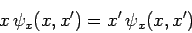(277)

for all. Consider the Dirac delta-function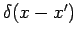. We can write(278)

sinceis only non-zero infinitesimally close to. Evidently,is proportional to. Let us make the constant of proportionality unity, so that(279)

Now, it is easily demonstrated that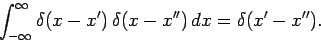(280)

Hence,satisfies the orthonormality condition(281)

This condition is analogous to the orthonormality condition (263) satisfied by square-integrable eigenstates. Now, by definition,satisfies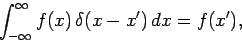(282)

where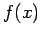is a general function. We can thus write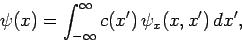(283)

where, or(284)

In other words, we can expand a general wavefunction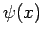as a linear combination of the eigenstates,, of the displacement operator. Equations (283) and (284) are analogous to Eqs. (261) and (264), respectively, for square-integrable eigenstates. Finally, by analogy with the results in Sect. 4.9, the probability density of a measurement ofyielding the valueis, which is equivalent to the standard result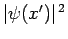. Moreover, these probabilities are properly normalized providedis properly normalized [cf., Eq. (265)]: i.e.,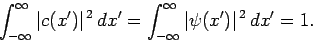(285)

Finally, if a measurement ofyields the valuethen the system is left in the corresponding displacement eigenstate,, immediately after the measurement: i.e., the wavefunction collapses to a spike-function'',, as discussed in Sect. 3.16.

Now, an eigenstate of the momentum operator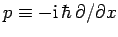corresponding to the eigenvalue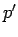satisfies(286)

It is evident that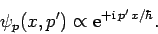(287)

Now, we require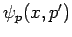to satisfy an analogous orthonormality condition to Eq. (281): i.e.,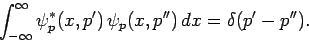(288)

Thus, it follows from Eq. (210) that the constant of proportionality in Eq. (287) should be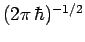: i.e.,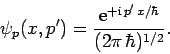(289)

Furthermore, according to Eqs. (202) and (203),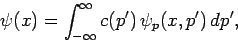(290)

where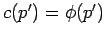[see Eq. (203)], or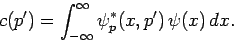(291)

In other words, we can expand a general wavefunctionas a linear combination of the eigenstates,, of the momentum operator. Equations (290) and (291) are again analogous to Eqs. (261) and (264), respectively, for square-integrable eigenstates. Likewise, the probability density of a measurement ofyielding the resultis, which is equivalent to the standard result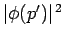. The probabilities are also properly normalized providedis properly normalized [cf., Eq. (221)]: i.e.,(292)

Finally, if a mesurement ofyields the valuethen the system is left in the corresponding momentum eigenstate,, immediately after the measurement.Next: Stationary States Up: Fundamentals of Quantum Mechanics Previous: Measurement
Richard Fitzpatrick 2010-07-20